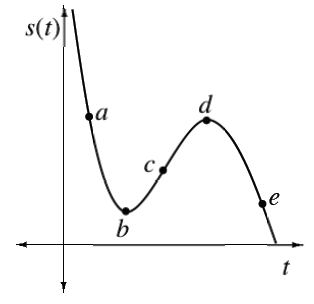### Home > APCALC > Chapter 4 > Lesson 4.1.3 > Problem4-36

4-36.

Using the distance vs. time graph at right, determine if the velocity is positive, negative, or zero at each labeled point on the graph.

Since the slope at a point is the same as the slope of the tangent line at that point, draw small tangent lines. Use their slopes to answer these questions.